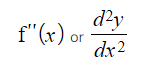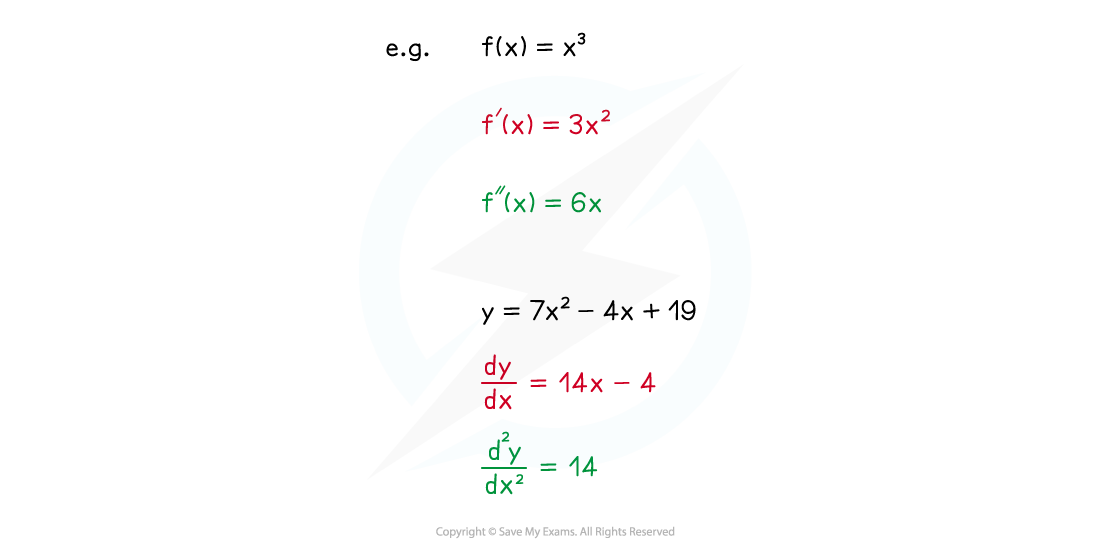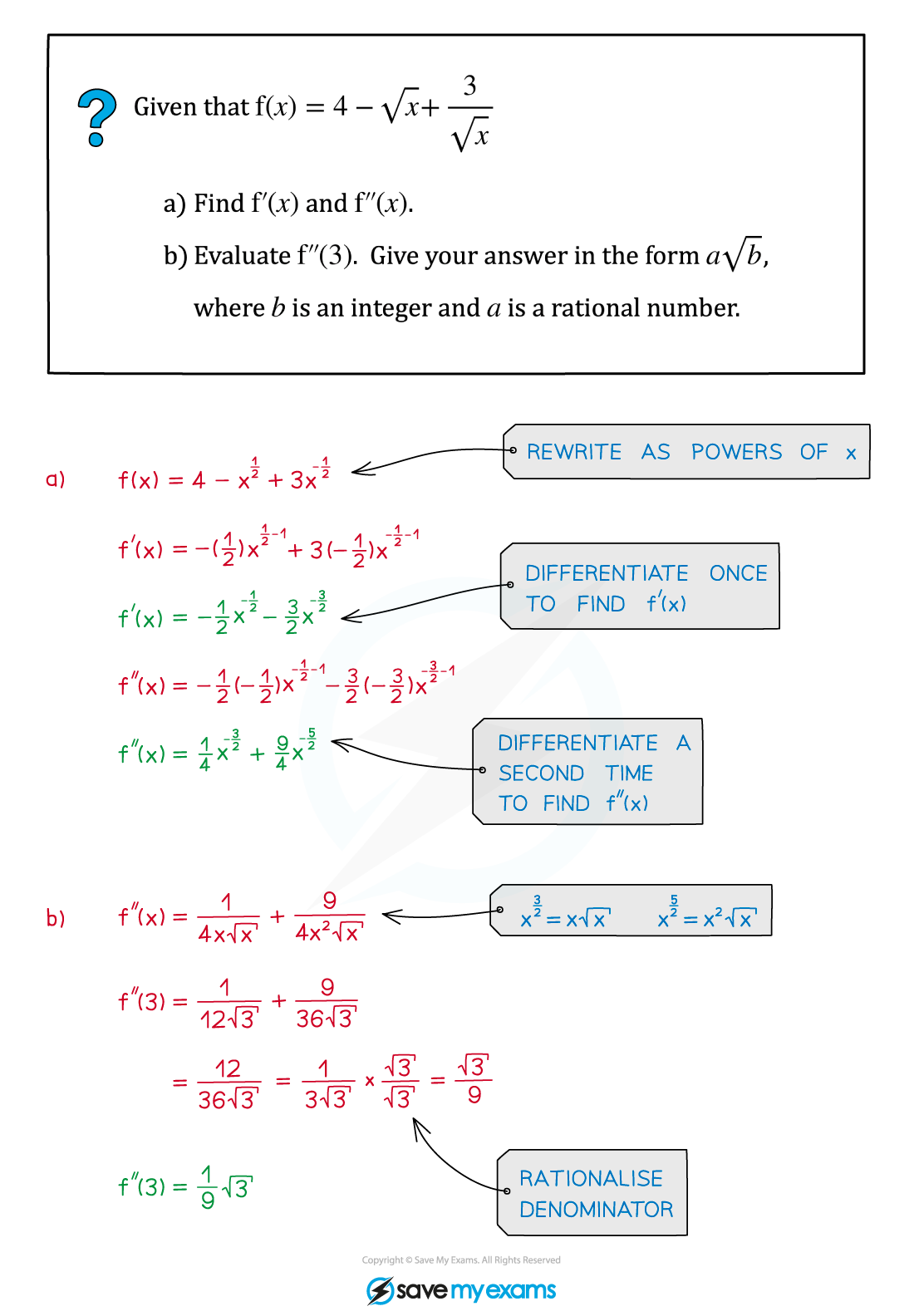# AQA A Level Maths: Pure复习笔记7.2.3 Second Order Derivatives

### Second Order Derivatives

#### What is the second order derivative of a function?

• If you differentiate the derivative of a function (ie differentiate the function a second time) you get the second order derivative of the function
• For a function y = f(x), there are two forms of notation for the second derivative (or second order derivative)• Note the positions of the power of 2's in the second version• The second order derivative can also be referred to simply as the second derivative
• Similarly, the 'regular' derivative can also be referred to as either the first order derivative or the first derivative
• The second order derivative gives the rate of change of the gradient function (ie of the first derivative) – this will be important for identifying the nature of stationary points

#### Exam Tip

• When finding second derivatives be especially careful with functions that have negative or fractional powers of x (see Worked Example below).
• Mistakes made with fractions or negative signs can build up as you calculate the derivative more than once.

#### Worked Example